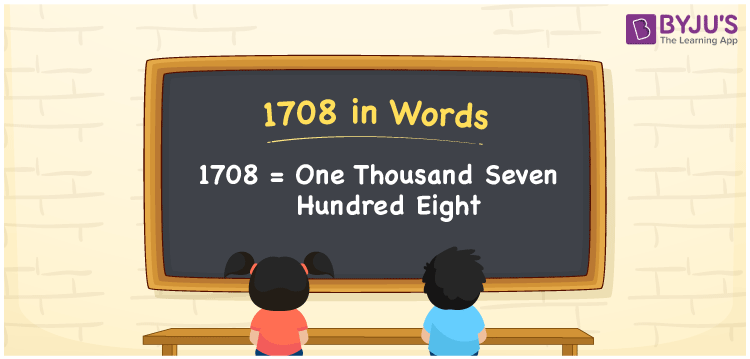The number 1708 in words can be expressed as One Thousand Seven Hundred Eight. For instance, the cost of a shirt is Rs. 1708, then you can say, “The cost of a shirt is Rupees One Thousand Seven Hundred Eight”. 1708 is a cardinal number as it denotes a certain amount. In this article, let us learn how to write the number name for 1708 using a place value system and its interesting facts in detail.

 1708 in Words One Thousand Seven Hundred Eight One Thousand Seven Hundred Eight in numerical form 1708

## 1708 in English Words

We generally write the numbers in words using the letters of the English alphabet. Hence, the number 1708 in English is written as One Thousand Seven Hundred Eight.## How to Write 1708 in Words?

Let us make a table of 4 columns to indicate the place value chart for 1708 since it is a four-digit number. Following is the place value chart for 1708.

 Thousands Hundreds Tens Ones 1 7 0 8

Therefore, we can write the expanded form as:

1 x Thousand + 7 x Hundred + 0 x Ten + 8 x One

= 1 x 1000 + 7 x 100 + 0 x 10 + 8 x 1

= 1000 + 700 + 0 + 8

= 1000 + 700 + 8

= 1708

= One Thousand Seven Hundred Eight

Hence, 1708 in words is written as One Thousand Seven Hundred Eight

Interesting way of writing 1708 in words

1 = One

17 = Seventeen

170 = One Hundred and Seventy

1708 = One Thousand Seven Hundred Eight

Thus, the word form of the number 1708 is One Thousand Seven Hundred Eight

1708 is a natural number that is one less than 1709 and one greater than 1707

• 1708 in words – One Thousand Seven Hundred Eight
• Is 1708 an odd number? – No
• Is 1708 an even number? – Yes
• Is 1708 a perfect square number? – No
• Is 1708 a perfect cube number? – No
• Is 1708 a prime number? – No
• Is 1708 a composite number? – Yes

## Frequently Asked Questions on 1708 in Words

Q1

### How to write 1708 in words?

We can write 1708 in words as One Thousand Seven Hundred Eight.
Q2

### Simplify 1000 + 708, and express in words.

Simplifying 1000 + 708, we get 1708. Thus, the number 1708 in words is One Thousand Seven Hundred Eight.
Q3

### What is the place value of 1 in 1708?

The place value of 1 in 1708 is Thousands.